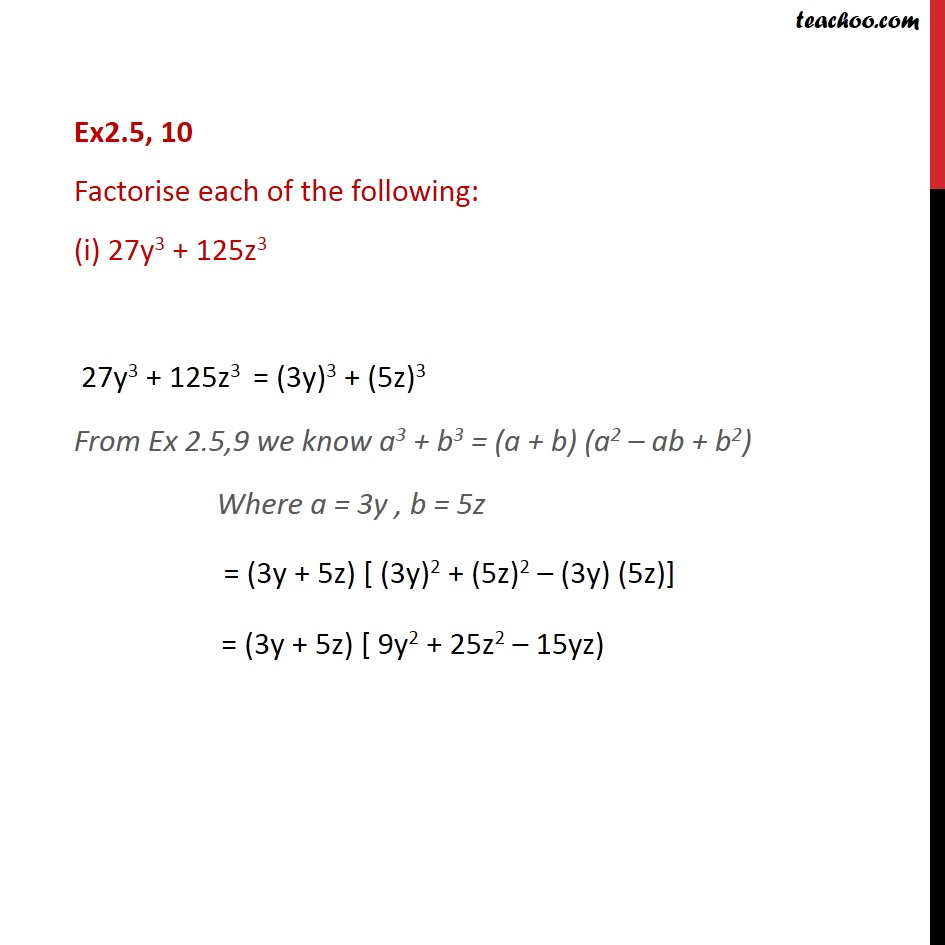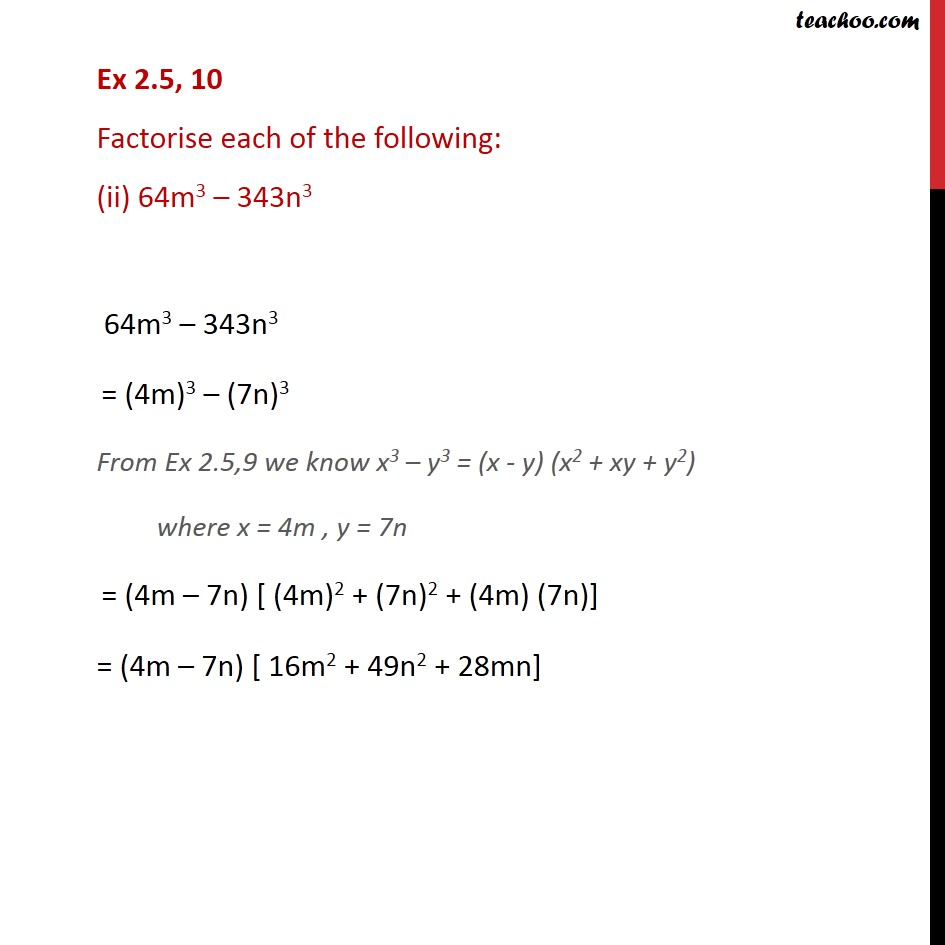Learn all Concepts of Polynomials Class 9 (with VIDEOS). Check - Polynomials Class 91. Chapter 2 Class 9 Polynomials
2. Serial order wise
3. Ex 2.5

Transcript

Ex2.5, 10 Factorise each of the following: (i) 27y3 + 125z3 27y3 + 125z3 = (3y)3 + (5z)3 From Ex 2.5,9 we know a3 + b3 = (a + b) (a2 – ab + b2) Where a = 3y , b = 5z = (3y + 5z) [ (3y)2 + (5z)2 – (3y) (5z)] = (3y + 5z) [ 9y2 + 25z2 – 15yz) Ex 2.5, 10 Factorise each of the following: (ii) 64m3 – 343n3 64m3 – 343n3 = (4m)3 – (7n)3 From Ex 2.5,9 we know x3 – y3 = (x - y) (x2 + xy + y2) where x = 4m , y = 7n = (4m – 7n) [ (4m)2 + (7n)2 + (4m) (7n)] = (4m – 7n) [ 16m2 + 49n2 + 28mn]

Ex 2.5

Chapter 2 Class 9 Polynomials
Serial order wise